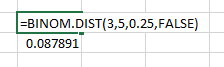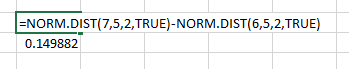# How to compute probabilities from a distribution (in Excel)

See all solutions.

There are many famous continuous probability distributions, such as the normal and exponential distributions. How can we get access to them in software, to compute the probability of a value/values occurring?

## Solution

If the probability distribution is a common discrete distribution, you can simply use a built-in function from Excel’s set of statistical functions to compute any probability from it.

For example, to find the probability that a binomial random variable with p=0.25 yields 3 successes in 5 trials, you can use =BINOM.DIST(3,5,0.25,FALSE). The final parameter, FALSE, tells Excel you are asking only about 3 successes, not the cumulative probability of up to 3 successes.For other discrete random variables, see the Excel help on POISSON.DIST and HYPGEOM.DIST.

If the probability distribution is a common continuous distribution, you must ask about the probability of a random value falling in a certain range. You do so by subtracting two outputs of the cumulative distribution function (CDF).

For example, to find the probability that a normal random variable with mean 5 and standard deviation 2 falls in the interval [6,7], you can use =NORM.DIST(7,5,2,TRUE)-NORM.DIST(6,5,2,TRUE). Notice:

• It is important to subtract the lower end of the interval from the higher end, not the other way around. (If your probability comes out negative, you have it backwards.)

• The final parameter, TRUE, tells Excel you are using the CDF of the distribution. If you use FALSE instead, you will get a wrong answer.For other continuous random variables, see the Excel help on BETA.DIST, CHISQ.DIST, F.DIST, GAMMA.DIST, LOGNORM.DIST, and T.DIST.